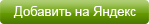# Виртуальная лаборатория ВиртуЛаб

 Меняя параметры, пользователь видит изменения в 3D среде как результат своих действий.

# The job purpose: experimentally to research interconnections between a strength of current, power and a resistance of explorers at their series connection.

### The equipment, gauges: 1) the power supply; 2) explorers of a particular resistance on a jamb; 3) a key; 4) the voltage metre; 5) the ampermeter.

JOB COURSE

1. Collect the electric circuit schema. Gauge a strength of current, having switched on the ampermeter at first between a radiant and one of explorers (I1), then between a radiant and other explorer (I2), and then between explorers (I3). Write in table 1 observed data.

2. Gauge power at first on one of explorers (U1), then on other explorer (U2), and then on both explorers (U). Outcome write in table 1.

3. Compute a resistance of the first explorer (R1), the second explorer (R2), discover their total; write results in table 1.

4. Compute a resistance of two explorers R, knowing power on them and a strength of current in a chain; write a result in table 1.

5. Draw outputs.

View job course

Run Consecutive bonds of explorers with Skockwave Player### + ФизикаВиртуальные лабораторные работы по Физике